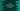# How to clone or copy a Vector in Java## Introduction :

In this tutorial, we will learn how to clone or copy a vector object in Java. The program will take user inputs to create one vector and then it will clone the vector to a different variable.

Vector is like a dynamic array in Java. Arrays are fixed. You can’t add extra items to an array. But vectors are of variable size. You can add as many items you want. The size of the vector will increase whenever you keep adding items to it.

Our program will first ask the user to enter the count of elements of the vector. It will then take the inputs of each element of the vector using a loop. Finally, it will clone the vector to a different variable and print out the result.

### Example Java Program :

``````import java.util.Scanner;
import java.util.Vector;

public class Example {
public static void main(String[] args) {
//1
int count;

//2
Scanner s = new Scanner(System.in);
Vector vector = new Vector<>();

//3
System.out.println("Enter total number of elements you want to add : ");
count = s.nextInt();

//4
for (int i = 0; i < count; i++) {
System.out.print("Enter string for position " + (i + 1) + " : ");
}

//5
Vector cloneVector = (Vector) vector.clone();

//6
System.out.println("New vector is : ");

for (Object aCloneVector : cloneVector) {
System.out.println(aCloneVector);
}
}
}``````

### Explanation :

The commented numbers in the above program denote the step numbers below :

1. Create one integer variable count to store the total size of the vector.
2. Create one Scanner variable s to read the user input. Also, create one Vector vector to hold string inputs.
3. Ask the user to enter the total size of the vector. Read the user input value using the Scanner s and store it in count variable.
4. Now, run one for loop to take the inputs for the vector from the user. On each iteration, read the user input and add it to the vector using add() method. We are reading the user input value using next() method.
5. This step is used for cloning the vector. For cloning, we have one built-in method called clone(). This new vector is stored in the cloneVector variable. Note that we need to cast the new value to a Vector.
6. Finally, print out the new vector to the user. We are using one for each loop to print out the content of the newly created vector.

### Sample Output :

``````Enter total number of elements you want to add :
3
Enter string for position 1 : Hello
Enter string for position 2 : World
Enter string for position 3 : !!
New vector is :
Hello
World
!!

Enter total number of elements you want to add :
2
Enter string for position 1 : 1
Enter string for position 2 : 1
New vector is :
1
1``````

### Conclusion :

Cloning a vector is easy using its built-in clone method. In this example, we have learned how to create a vector using user inputs, how to clone a vector and also how to loop through all elements of the vector. Try to run the example program we have shown above and drop one comment below if you have any queries.